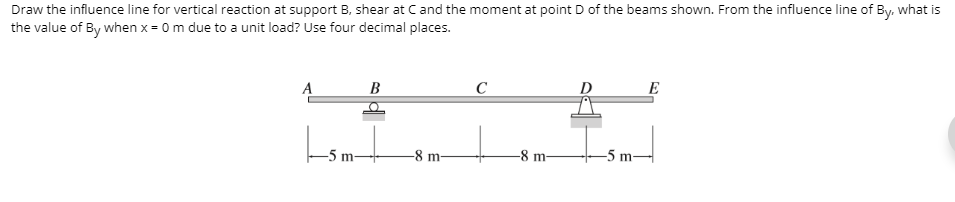# Question Draw the influence line for vertical reaction at support B, shear at Cand the moment at point of the beams shown. From the influence line of By, what is the value of By when x = 0 m due to a unit load? Use four decimal places. A B с E -5 m -8 m -8 m -5 mLBYSDK The Asker · Civil EngineeringTranscribed Image Text: Draw the influence line for vertical reaction at support B, shear at Cand the moment at point of the beams shown. From the influence line of By, what is the value of By when x = 0 m due to a unit load? Use four decimal places. A B с E -5 m -8 m -8 m -5 m
More
Transcribed Image Text: Draw the influence line for vertical reaction at support B, shear at Cand the moment at point of the beams shown. From the influence line of By, what is the value of By when x = 0 m due to a unit load? Use four decimal places. A B с E -5 m -8 m -8 m -5 m